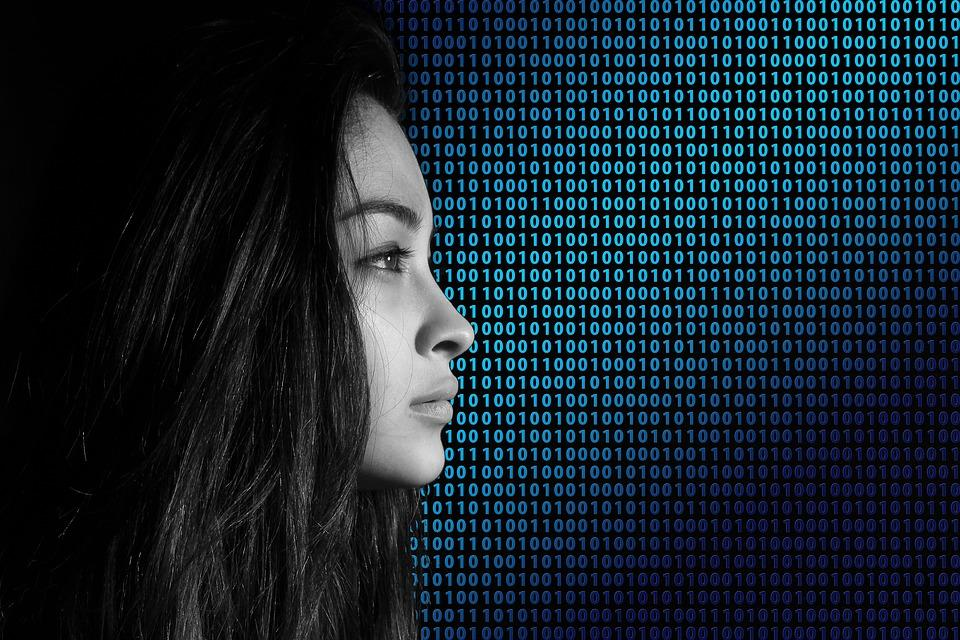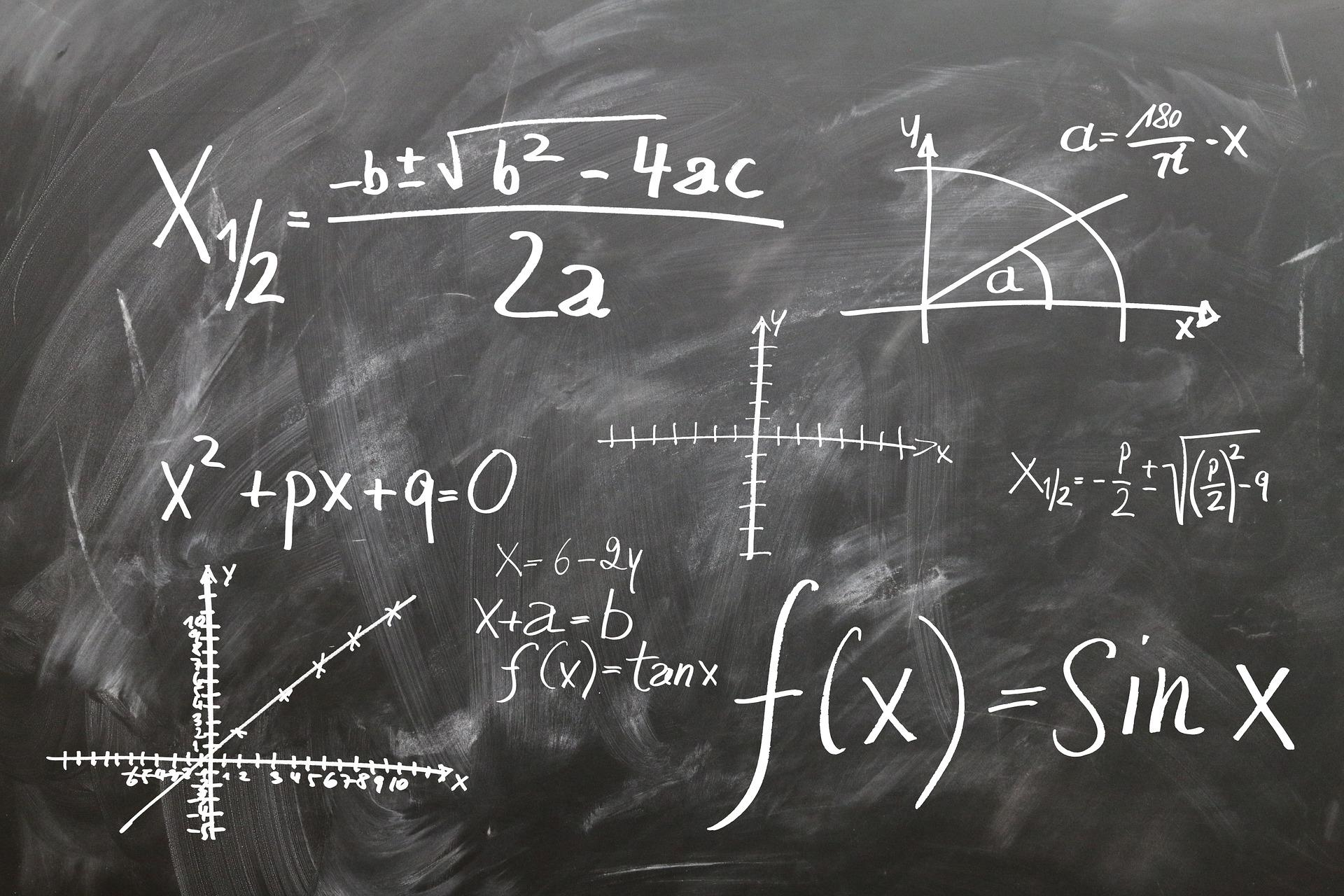As a new field of study, computer science covers topics such as theoretical studies of computation, algorithms, and information. With a computer science degree from any of the best schools, colleges, or universities, you’ll have a bright, prosperous future (Source: Pixabay)

Unlike practical subjects like political science or natural science – which analyzes the real-world – computer science is new and much more challenging to absorb.

It’s a fascinating field; hence, students flock to enroll in computer science degree programs every year despite its complex nature.

On the other hand, some skeptics still wonder if computer science is a good major to take. The answer is yes!

Having a computer science degree from a reputable college or university means you’ll have several exciting opportunities in the future.

And if you’re wondering what you can do with a computer science degree, here are some options that you can take after graduation:

• Software engineer
• Systems analyst
• Application analyst
• Applications developer
• Data analyst
• Cyber-security analyst
• Game designer
• Game developer
• IT consultant
• Web developer
• Web designer
• UX designer
• Information systems manager
• Forensic comp analyst
• Network engineer
• Nanotechnologist
• Telecommunications researcher
• IT trainer
• Supply-chain manager
• Multimedia programmer
• Technical writer
• Product manager
• Full-stack developer
• Cloud computing engineer
• QA/Test engineer

However, if you want to become an accomplished computer scientist, you may require an advanced or intermediate understanding of other subjects, including mathematics.

But before we delve into the particulars, you must understand that not every computer programmer uses mathematics every day.

As a matter of fact, some programmers never even have to use it. Nevertheless, mathematics is helpful for two good reasons:

• Many computer programmers do use mathematics daily, which makes the subject an essential requirement for a computer science degree
• Mathematics can help develop the fundamental logic required to work in computer science

Still wondering why math is so important for computer programming? Read on!The best tutors availableTobiah
\$40
/h1st lesson free!5 (9 reviews)
Benjamin
\$50
/h1st lesson free!Maud
\$40
/h1st lesson free!5 (2 reviews)
William
\$25
/h1st lesson free!5 (2 reviews)
Lourdes
\$50
/h1st lesson free!5 (1 reviews)
Aubrey
\$60
/h1st lesson free!5 (16 reviews)
Andrea
\$35
/h1st lesson free!Thomas
\$50
/h1st lesson free!5 (2 reviews)
Wendy k
\$28
/h1st lesson free!5 (5 reviews)
Stanley
\$40
/h1st lesson free!5 (1 reviews)
Richard
\$75
/h1st lesson free!Tom
\$30
/h1st lesson free!Tobiah
\$40
/h1st lesson free!5 (9 reviews)
Benjamin
\$50
/h1st lesson free!Maud
\$40
/h1st lesson free!5 (2 reviews)
William
\$25
/h1st lesson free!5 (2 reviews)
Lourdes
\$50
/h1st lesson free!5 (1 reviews)
Aubrey
\$60
/h1st lesson free!5 (16 reviews)
Andrea
\$35
/h1st lesson free!Thomas
\$50
/h1st lesson free!5 (2 reviews)
Wendy k
\$28
/h1st lesson free!5 (5 reviews)
Stanley
\$40
/h1st lesson free!5 (1 reviews)
Richard
\$75
/h1st lesson free!Tom
\$30
/h1st lesson free!

## 5 Kinds Of Mathematics Used In A Computer Science DegreeMany ask whether computer science is a good major, offering decent future prospects or not. Believe it or not, its cross-discipline nature makes it more beneficial than you think. There are as many as five kinds of maths employed in computer science (Source: Pixabay)

Computer programming is one of the unique practical fields. While its complex languages will take some time to learn, the simpler ones take much less time to master.

For more complex computer science careers, you should be armed with the knowledge of calculus, binary maths, discrete maths, etc.

Moreover, since mathematics is an area of study you can never completely learn, you’ll need to take several courses before heading to advanced computer science classes.

Therefore, before starting your computer science degree, brush up on:

### Binary Maths

Binary mathematics is the focal point of computer functions and is readily used in computer programming. It’s used to represent every number inside the computer.

In addition to that, the ‘binary number system’ is a substitute for the ‘decimal system.’ Thus, using it streamlines computer design.

Moreover, simple mathematical functions are vital for low-level hardware programming. Therefore, knowing ‘hexadecimal number system’ assists with multiple programming operations such as setting/changing the colors of items.

Basic arithmetic is also utilized in computer science. For example, division, multiplication, subtraction, and addition are used in almost all written programs.

### College Algebra

College algebra includes concepts such as:

• Operations
• Linear equations
• Exponents
• Factoring
• Polynomials
• Rational expressions
• Rectangular coordinates
• Proportions
• Ratios

Moreover, it emphasizes algebraic relationships, functions, and graphs. In addition to that, students learn to graph various algebra operations.

It’s employed in computer programming to create software and algorithms to work along with different mathematical objects.

Apart from that, algebra is also utilized to design equations employed in numerical programming and scientific computations.

### Statistics

Statistics is another kind of maths employed in a computer science degree. It utilizes quantified models, synopses, and representations for a given collection of actual studies or experimental data.

It studies methodologies for obtaining, reviewing, evaluating, and forming conclusions from available data.

Furthermore, some of its key measures include:

• Skewness
• Averages
• Regression analysis
• Variance analysis
• Kurtosis
• Variance

Statistics play a crucial role in computer programming as it’s used for speech recognition, data mining, data compression, artificial intelligence, traffic modeling, and image and vision analysis. Apart from that, it is utilized for simulations as well.

Therefore, a statistics background is required to understand the statistical properties and algorithms of computer programming.

### Calculus

Calculus is the kind of maths that studies continuous change. Not only does it examine alterations, but it also measures the rate at which it occurs.

It manages the properties of derivatives and integrals of functions. Additionally, calculus is further divided into two types: integral calculus and differential calculus.

Differential calculus studies the “rates of change” of the quantity, whereas integral calculus deals with the application and theory of integrals.

To put it simply, the latter deals with total value or size, such as areas, volumes, and lengths.

This field of mathematics is also utilized in a wide array of programming areas such as making visuals or graphs, simulations, coding-in applications, problem-solving applications, analysis and design of algorithms, and making statistic solvers.

### Discrete Mathematics

Discrete mathematics analyzes objects that can be portrayed finitely. It involves a vast majority of topics, all of which can answer numerous tangible inquiries.

It includes concepts such as:

• Number theory
• Logic
• Probability
• Counting
• Recurrences
• Graph theory

Discrete mathematics offers a crucial foundation for every area of computer programming. Furthermore, it’s used in many different areas like:

• Algorithms
• Computer architecture
• Computer systems
• Functional programming
• Databases
• Machine learning
• Distributed systems
• Networks
• Computer security
• Operating systems

The problem-solving techniques taught in this kind of mathematics are essential for composing complex computer programming software.

Hence, you should take up calculus if you want a future in computer science.

## 4 Crucial Reasons Why Mathematics Is So Important For Computer ProgrammingMaths is essential for computer programming; that’s why high school students interested in computer science should learn and become experts in mathematics (Source: Pixabay)

High school students interested in computer science should know very well that the ability to do math is imperative for a computer science degree.

Not only does mathematics help you get a better CGPA in your computer science degree, but it will also improve your performance in other subjects.

Here are four reasons why mathematics is crucial for computer programming:

### Mathematics Teaches Communication And Understanding Through An Abstract Language

Computer science has approx. 700 programming languages. And there’s one thing common among all: they’re highly abstract.

Through syntax, you’ll have to represent specific commands, processes, and visuals via symbols, single words, and punctuation.

Students with little to no experience communicating or thinking in an abstract language will find computer science terrifying.

However, an abstract programming language is much like what students study in a mathematics class at school.

From complex number representations to simple equalities, learning maths teaches learners to comprehend, communicate, formulate thoughts, and read abstract languages.

Of course, computer science languages and mathematical languages aren’t the same; however, the experience of using any ‘abstract language’ gives beginners an edge.

### Mathematics Teaches How You Should Work With An Algorithm

Believe it or not, the algorithm is one of the most talked-about terminologies in the tech scene. To put it simply, the algorithm is the depiction of any process in a shape which can be applied to different (new) problems.

Though you will hear this word throughout your computer science degree, many learners are first introduced to algorithms in maths. For instance, consider the following equation:

5+x = 7

Pupils in schools learn to seek an unknown addend by subtracting a known addend from the sum-total. This is called an algorithm, one that school pupils promptly learn to use in new mathematical problems.

### Mathematics Teaches Learners How To Evaluate Their Work

Whenever there’s a load of programming to do, the chances are that every computer programmer will make an error.

Therefore, computer programmers should know how to evaluate a problem, assess their daily work, and fix problems.

Mathematics is among the few fields where students evaluate their day-to-day work like this. For example, a pupil may face the question: “how much does a kitten weigh.” And with the values they have, they could end up getting the answer “150 pounds.”

They will assess their process to find the error and fix it, such as they may have overlooked converting to pounds from ounces. Mathematics, in short, helps students develop analytical tendencies.

### Apart From General Skills, A Computer Science Degree Still Include Lots Of Mathematics

Apart from the traditional skills necessary for computer programming, the figures and facts of mathematics are also important.

As computer science engages becomes more relevant, the importance of quantifiable and measurable data also grows.

For instance, to make self-driving vehicles, the equations used to calculate turning angles, suitable distance from other cars, and acceleration should be spot on.

So, if you want to learn more about computer science, you’ll need a good deal of mathematical skills and knowledge.

But more importantly, if you want to have a successful career, you must possess the ability to process things mathematically.

## Take Maths Lessons To Improve Your Chances Of Getting A Computer Science Degree

One of the most effective ways to get better prospects in computer programming is by learning mathematics – as it plays a significant role in a computer science degree.

Alongside extra-curricular research, the best way to study maths is by taking private maths lessons in your hometown.

Choose Superprof – the best online and in-house tutoring platform in the US, providing the best and most convenient education to learners.

They have a wide array of experienced math tutors ready to provide their services. So why wait anymore? Sign up to Superprof and become a math expert!The platform that connects tutors and students4.00 (2 rating(s))Loading...